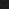JavaPerspective.com  >   Beginner Tutorials  >   3. Java Basics  >   3.5. Operators and expressions

3.5. Operators and expressions
Last updated: 23 January 2013.

3.5.1. Expressions

Expressions are built with constants, variables, operators and method calls. An expression evaluates to a single value and is part of a statement.

For example, if you have declared and initialized an integer variable named x like this:

int x = 10;

then you can use x as an expression (it evaluates to a single value since it is a variable with its own value). The constant 100 is also an expression. Together, those two expressions can make up a larger expression that still evaluates to a single value:

x + 100

That larger expression can also be part of an even larger expression:

(x + 100) * 3

The above expression also evaluates to a single value and it can be part of the following statement:

int y = (x + 100) * 3;

3.5.2. Operators

### 3.5.2.1. Assignment operator

Operator Use Purpose
= op1  =  op2 Replaces the value of op1 with the value of op2

### 3.5.2.2. Arithmetic operators that require at least two operands

Operator Use Purpose
+ op1  +  op2 Adds numbers, concatenates Strings
- op1  -  op2 Subtracts numbers
* op1  *  op2 Multiplies numbers
/ op1  /  op2 Divides numbers
% op1  %  op2 Gets the remainder of the division of two numbers

### 3.5.2.3. Arithmetic operators that require only one operand

Operator Use Purpose
! ! op Inverts the value of a boolean, i.e., returns true if op is false
+ + op Indicates that op is a positive number
- - op Indicates that op is a negative number
++ ++ op Increments op by 1 before using it to evaluate the larger expression
++ op ++ Increments op by 1 after having used it to evaluate the larger expression
-- -- op Decrements op by 1 before using it to evaluate the larger expression
-- op -- Decrements op by 1 after having used it to evaluate the larger expression

### 3.5.2.4. Conditional and relational operators

Operator Use Purpose
< op1  <  op2 Returns true if op1 is less than op2
<= op1  <=  op2 Returns true if op1 is less than or equal to op2
> op1  >  op2 Returns true if op1 is greater than op2
>= op1  >=  op2 Returns true if op1 is greater than or equal to op2
== op1  ==  op2 Returns true if op1 is equal to op2
!= op1  !=  op2 Returns true if op1 is not equal to op2
&& op1  &&  op2 Returns true if both op1 and op2 are true, evaluates op2 only if op1 is true
& op1  &  op2 Returns true if both op1 and op2 are true, always evaluates op1 and op2
|| op1  ||  op2 Returns true if op1 or op2 is true, evaluates op2 only if op1 is false
| op1  |  op2 Returns true if op1 or op2 is true, always evaluates op1 and op2

### 3.5.2.5. Bitwise operators

Operator Use Purpose
& op1  &  op2 Bitwise and
| op1  |  op2 Bitwise or
^ op1  ^  op2 Bitwise xor
~ ~  op Bitwise complement (inverts the bits)
<< op1  <<  op2 shift bits of op1 left by op2
>> op1  >>  op2 shift bits of op1 right by op2
>>> op1  >>>  op2 shift bits of op1 right by op2 (unsigned)

### 3.5.2.6. Type checking operator

Operator Use Purpose
instanceof op1  instanceof  op2 Returns true if op1 and op2 have the same type (op1 and op2 must be objects)

The next tutorial will show you how to use the Java operators.

You are here :  JavaPerspective.com  >   Beginner Tutorials  >   3. Java Basics  >   3.5. Operators and expressions
Next tutorial :  JavaPerspective.com  >   Beginner Tutorials  >   3. Java Basics  >   3.6. Java operators examples